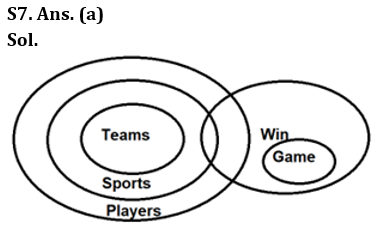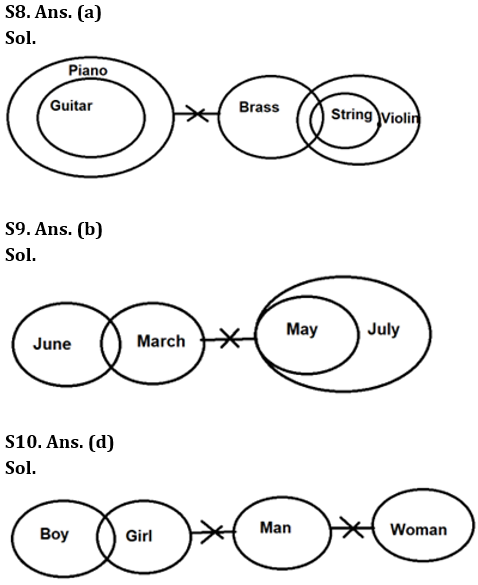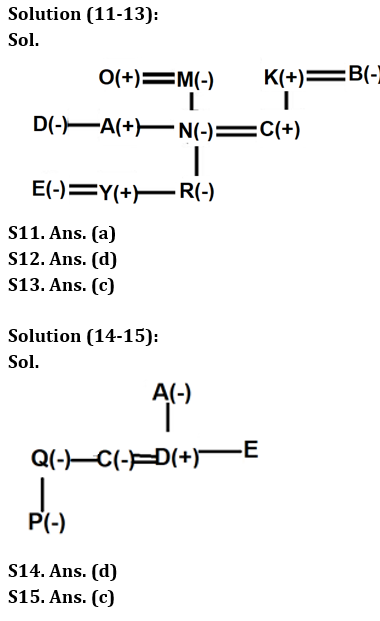Latest Banking jobs   »

# Reasoning Quiz For RBI Grade B Phase 1 2023-22nd March

Directions (1-5): When a word and number arrangement machine is given an input line of words and numbers, it arranges them following a particular rule. The following is an illustration of input and rearrangement.

Input: naughty 46 dynamic 49 provide 38 advance 78 minus 65
Step 1: advance 24 naughty 46 dynamic 49 provide 78 minus 65
Step II: dynamic 24 advance 24 naughty 49 provide 78 minus 65
Step III: minus 36 dynamic 24 advance 24 naughty provide 78 65
Step IV: naughty 30 minus 36 dynamic 24 advance 24 provide 78
Step V: provide 56 naughty 30 minus 36 dynamic 24 advance 24
Step VI: provide 11 naughty 03 minus 09 dynamic 06 advance 06
And step VI is the last step of the rearrangement. As per the rules followed in the above step, rearrange the given input and answer the related questions.

Input: roast 45 glue 76 standard 28 drawing 88 problem 37

Q1. Which of the following is step V of the given input after the rearrangement?
(a) roast 42 problem 20 glue 21 drawing 16 standard 88
(b) standard 10 roast 06 problem 02 glue 03 drawing 07
(c) standard 64 roast 42 problem 20 glue 21 drawing 16
(d) glue 21 drawing 16 roast 45 76 standard 88 problem
(e) None of these

Q2. In which step of the given input, ‘64 roast 42 problem’ will appear in the same sequence after the rearrangement?
(a) Step III
(b) Step V
(c) Step VI
(d) Step IV
(e) None of these

Q3. What is the difference between 5th number from the right end and 4th number from the left end in step IV of the given input after the rearrangement?
(a) 26
(b) 25
(c) 21
(d) 28
(e) 27

Q4. Which of the following element is 5th from the left end in step II of the given input?
(a) Roast
(b) 16
(c) Glue
(d) 45
(e) Drawing

Q5. Which of the following element does not appear in step V of the given input?
(a) 42
(b) 20
(c) Glue
(d) 88
(e) Drawing

Direction (6-10): In the questions below, three statements are given followed by the conclusions. You have to take the given statements to be true even if they seem to be at variance with commonly known facts. Read all the conclusions and then decide which of the given conclusions logically follows from the given statements disregarding commonly known facts.

Q6. Statements: Only Sum are Divide.
Some Minus are Sum.
Only a few Minus are Number.
No Sum are Product.
Conclusions: I. A few Number being Divide is a possibility
II. Some Minus are not Product
(a) If only conclusion I follows
(b) If only conclusion II follows
(c) If either conclusion I or conclusion II follows
(d) If both conclusions I and II follow
(e) If neither conclusion I nor conclusion II follows

Q7. Statements: All Teams are Sports.
All Sports are Players.
Only Win are Game.
A few Sports are Win.
Conclusions: I. At least Some Sport are Team
II. At least Some Teams are Win
(a) If only conclusion I follows
(b) If only conclusion II follows
(c) If either conclusion I or conclusion II follows
(d) If both conclusions I and II follow
(e) If neither conclusion I nor conclusion II follows

Q8. Statements: Only Piano are Guitar.
No Piano is Brass.
Only a few Brass are Strings.
All Strings are Violin.
Conclusions: I. A few Strings being Guitar is not a possibility
II. No Brass is Violin
(a) If only conclusion I follows
(b) If only conclusion II follows
(c) If either conclusion I or conclusion II follows
(d) If both conclusions I and II follow
(e) If neither conclusion I nor conclusion II follows

Q9. Statements: No March is May.
Only a few June is March.
All May is July.
Conclusions: I. Some March is July.
II. All March being June is a possibility.
(a) If only conclusion I follows
(b) If only conclusion II follows
(c) If either conclusion I or conclusion II follows
(d) If both conclusions I and II follow
(e) If neither conclusion I nor conclusion II follows

Q10. Statements: Only a few boy is girl.
No girl is man.
No man is woman.
Conclusions: I. Some woman being girl is a possibility
II. A few boy can never be man
(a) If only conclusion I follows
(b) If only conclusion II follows
(c) If either conclusion I or conclusion II follows
(d) If both conclusions I and II follow
(e) If neither conclusion I nor conclusion II follows

Direction (11-13): Read the following information carefully and answer the questions given below.
In a family of three-generation, there are eleven members i.e. A, N, C, B, M, K, R, Y, E, O and D in which four married couples are there. A is the brother-in-law of C. Only one child of M is married. K is the paternal grandfather of R. N is the daughter-in-law of B. E is the married female member of the family. C has no siblings. M has only one son. Y is the nephew of D. Y is not child of A. D is the sibling of N. R is the granddaughter of M. O is the married member of the family and belongs to the first generation of the family. M is the mother in-law of C.

Q11. What is the relation of Y with respect to O?
(a) Grandson
(b) Son
(c) Uncle
(d) Father
(e) None of these

Q12. How many female members are there in the family?
(a) More than six
(b) Five
(c) Cannot be determined
(d) Six
(e) None of these

Q13. Who among the following is the paternal grandmother of R?
(a) O
(b) K
(c) B
(d) Cannot be determined
(e) None of these

Direction (14-15): Read the following information carefully and answer the questions given below.
‘A @ B’ means ‘A is the sister of B’.
‘A # B’ means ‘A is the son of B’.
‘A % B’ means ‘A is the daughter of B’.
‘A * B’ means ‘A is the mother of B’.
‘A \$ B’ means ‘A is the wife of B’.
Now answer the following questions based on the above relations.

Q14. If the expression ‘P % Q @ C \$ D # A *E’ holds true, then which of the following statement is definitely false?
(a) D is the husband of C
(b) Q is the sister-in-law of D
(c) P is the niece of C
(d) E is the uncle of C
(e) None of these

Q15. If the expression ‘P % Q @ C \$ D # A *E’ holds true, then what is the relation of A with respect to C?
(a) Son-in-law
(b) Daughter-in-law
(c) Mother-in-law
(d) Father-in-law
(e) None of these

Solutions

Solution (1-5):
Sol. In the first step, the word, which comes first in dictionary order, is placed at the extreme left end. The smallest number is replaced by its product of digits and is placed 2nd from the extreme left end (just after the first word).
In the second step, the word, which comes second in dictionary order, is placed, at the extreme end. The 2nd smallest number is replaced by its product of digits and is placed 2nd from the extreme left end (both the elements are arranged just before the elements which are arranged in first step). This process continues for all the words and numbers.
In last step, only the digits of the number are added and words will remain same.

Input: roast 45 glue 76 standard 28 drawing 88 problem 37
Step 1: drawing 16 roast 45 glue 76 standard 88 problem 37
Step II: glue 21 drawing 16 roast 45 76 standard 88 problem
Step III: problem 20 glue 21 drawing 16 roast 76 standard 88
Step IV: roast 42 problem 20 glue 21 drawing 16 standard 88
Step V: standard 64 roast 42 problem 20 glue 21 drawing 16
Step VI: standard 10 roast 06 problem 02 glue 03 drawing 07

S1. Ans. (c)
S2. Ans. (b)
S3. Ans. (a)
S4. Ans. (a)
S5. Ans. (d)## FAQs

### What is the selection process of the Bank Clerk?

There are 4 sections in the RBI Grade B Phase 1 Exam i.e. English Language, General Awareness, Quantitative Aptitude & Reasoning.

#### Congratulations!Union Budget 2023-24: Free PDF